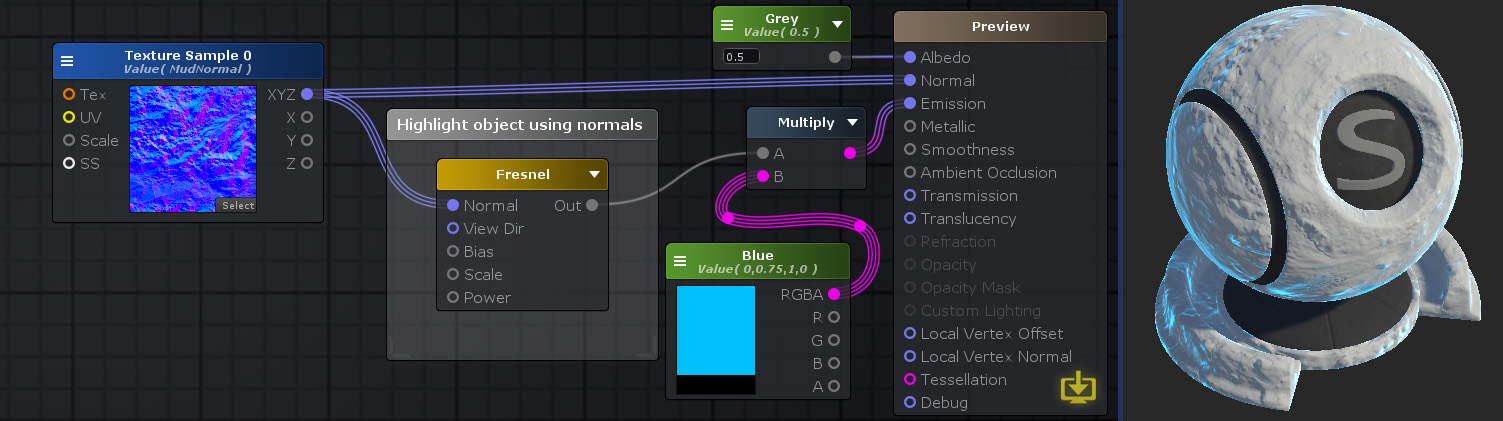## Fresnel Node

The Fresnel node outputs the result of a Fresnel effect. It defines how light behaves when it reaches an interface between two materials with different refractive indices, how much of it is reflected and refracted.
This node in particular deals with the reflection part and calculates the Fresnel Reflection Coefficient which is defined by the following expression ReflectionCoefficient = Bias + Scale x ( 1 + N.I )Power.
Each member of the equation can be modified except the I variable which defines an Incident vector from the camera to the object and is internally calculated by the node as the inverted World View Direction vector.Nodes used: Float, Texture Sample, Fresnel, Color, Multiply

Node Parameter Description Default Value
Normal Space Specifies in which coordinates space the normal vector is
• Tangent: Normal vector is in a tangential space coordinates
• World: Normal vector is in a world space coordinates
Tangent
Bias Defines the Bias variable of the Fresnel equation. Only visible if the respective input port is not connected. 0
Scale Defines the Scale variable of the Fresnel equation. Only visible if the respective input port is not connected. 1
Power Defines the Power variable of the Fresnel equation. Only visible if the respective input port is not connected. 5

Input Port Description Type
Normal Normal vector to be used. Surface world normal will be used if left unconnected. Float 3
Bias Defines the Bias variable of the Fresnel equation. Float
Scale Defines the Scale variable of the Fresnel equation. Float
Power Defines the Power variable of the Fresnel equation. Float